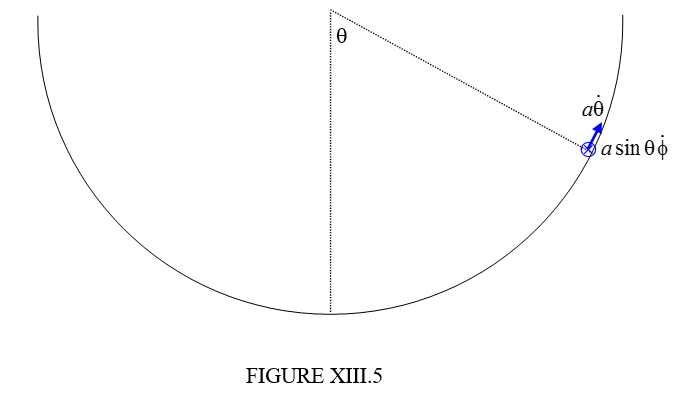Skip to main content
$$\require{cancel}$$

# 13.7: Slithering Soap in Hemispherical Basin

•• Contributed by Jeremy Tatum
• Emeritus Professor (Physics & Astronomy) at University of Victoria

Suppose that the basin is of radius a and the soap is subject to the holonomic constraint $$r=a$$ - i.e. that it remains in contact with the basin at all times. Note also that this is just the same constraint of a pendulum free to swing in three-dimensional space except that it is subject to the holonomic constraint that the string be taut at all times. Thus any conclusions that we reach about our soap will also be valid for a pendulum.

We’ll start with the newtonian approach, and I’ll draw in red the two forces on the soap, namely its weight and the normal reaction of the basin on the soap. Figure XIII.4We’ll make use of the expressions for the radial, meridional and azimuthal accelerations from Section 13.5 and we’ll write down the equations of motion in these directions:

Radial:

$mg\cos\theta-R=m(\ddot{r}-r\dot{\theta}^{2}-r\sin^{2}\theta\dot{\phi}^{2}). \label{13.7.1}$

Meridional:

$-mg\sin\theta=m(r\ddot{\theta}-2\dot{r}\dot{\theta}-r\sin\theta\cos\theta\dot{\phi}^{2}), \label{13.7.2}$

Azimuthal:

$0=m(2\dot{r}\dot{\phi}\sin\theta+2r\dot{r}\dot{\phi}\cos\theta+r\sin\theta\ddot{\phi}) \label{13.7.3}$

We also have the constraint that $$r=a$$ and hence that $$\dot{r}=\ddot{r}=0$$, after which these equations become

$mg\sin\theta-R=-ma(\dot{\theta}^{2}+\sin^{2}\theta\dot{\phi}^{2}), \label{13.7.4}$

$-g\sin\theta=a(\ddot{\theta}-\sin\theta\cos\theta\dot{\phi}^{2}), \label{13.7.5}$

$0=\sin\theta\ddot{\phi}+2\dot{\theta}\dot{\phi}\cos\theta. \label{13.7.6}$

These, then, are the newtonian equations of motion. If you still prefer the newtonian method to the lagrangian method, and you wish to integrate these and find expressions $$\theta$$, $$\phi$$ and $$R$$ separately, by all means go ahead and do so – but I’m now going to try the lagrangian approach.

Although Lagrange himself would not have drawn a diagram, we shall not omit that step – but instead of marking in the forces, we’ll mark in the velocity components, and then we’ll immediately write down expressions for the kinetic and potential energies. Indeed the first line of a lagrangian calculation is always “$$T=$$...”.$T=\frac{1}{2}ma^{2}(\dot{\theta}^{2}+\sin^{2}\theta\dot{\phi}^{2}) \label{13.7.7}$

$V=-mga\cos\theta+constant \label{13.7.8}$

Now apply Equation 13.4.13 in turn to the coordinates $$\theta$$ and $$\phi$$.

$$\theta$$:

$a\ddot{\theta}-a\sin\theta\cos\theta\dot{\phi}^{2}=-g\sin\theta. \label{13.7.9}$

$$\phi$$:

As for the conical basin, we see that $$\frac{\partial T}{\partial\phi}$$ and $$\frac{\partial V}{\partial\phi}$$ are both zero ($$\phi$$ is an "ignorable coordinate") and therefore $$\frac{\partial T}{\partial\dot{\phi}}$$ is constant and equal to its initial value. If the initial values of $$\dot{\phi}$$ and $$\theta$$ are $$\Omega$$ and $$\alpha$$ respectively, then

$\sin^{2}\theta\dot{\phi}=\sin^{2}\alpha\Omega. \label{13.7.10}$

This is merely stating that angular momentum is conserved.

We can easily eliminate $$\dot{\phi}$$ from Equations 13.4.9 and 13.4.10 to obtain

$\ddot{\theta}=k\cot\theta\csc^{2}\theta-\frac{g\sin\theta}{a}, \label{13.7.11}$

where

$k=\sin^{4}\alpha\Omega^{2}. \label{13.7.12}$

Write $$\ddot{\theta}$$ as $$\dot{\theta}\frac{d\dot{\theta}}{d\theta}$$ in the usual way and integrate to obtain the first space integral:

$\dot{\theta}^{2}=\frac{2g}{a}(\cos\theta-\cos\alpha)-k(\csc^{2}\theta-\csc^{2}\alpha). \label{13.7.13}$

The upper and lower bounds for $$\theta$$ occur when $$\dot{\theta}=0$$

Example. Suppose that the initial value of $$\theta$$ is $$\alpha$$ = 45o and that we start by pushing the soap horizontally ($$\dot{\theta}=0$$) at an initial angular speed $$\Omega$$ = 3 rad s-1, so that $$k$$ = 2.25 rad2 s-2. Suppose that the radius of the basin is $$a$$ = 1.96 m and that $$g$$ = 9.8 m s-2. You can then put $$\dot{\theta}=0$$ in Equation $$\ref{13.7.13}$$ and solve it for $$\theta$$. One solution, of course, is $$\theta=\alpha$$. We could find the other solution by Newton-Raphson iteration, or by putting $$\csc^{2}\theta=\frac{1}{(1-\cos^{2}\theta)}$$ and solving it as a cubic equation in $$\cos\theta$$. Alternatively, try this:

Let $$\frac{ak}{2g}=n,\quad\cos\theta=x,\quad\cos\alpha=c,$$

so that $$\csc^{2}\theta=\frac{1}{(1-x^{2})}$$ and $$\csc^{2}\alpha=\frac{1}{(1-c^{2})}$$.

The equation then becomes a quadratic equation in $$x$$, with solution

That is, $$x=\frac{-n+\sqrt{n^{2}-4(1-c^{2})[c(n+c)-1]}}{2(1-c^{2})}$$

I’ll leave you to re-write this in terms of what these quantities originally meant.